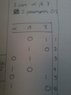# Need help with number of equiprobable outcomes

• controlswhiz

## Homework Statement

A subway train made up of $n$ cars is boarded by $r$ passengers $(r < n)$,
each entering a car completely at random. What is the probability of the
passengers all ending up in different cars?

## Homework Equations

$P(A) = \frac{N(A)}{N}$

$A$ - no more than one passenger enters any car

## The Attempt at a Solution

Part 1. Finding the total number of equiprobable outcomes $N$
The book says that the number of equiprobable outcomes $N$ is $n^r$.
I've set up a table (included in the attachment) with 3 cars and 2
passengers and came up with only 6 equiprobable outcomes. What
I don't understand is that the passenger will not be in two cars at
once so why would the solution be $N = n*n*...*n = n^r$.

EDIT:
Another book with the same problem with the same solution.

#### Attachments

•2.jpg
9.4 KB · Views: 345
Last edited:

## Homework Statement

A subway train made up of $n$ cars is boarded by $r$ passengers $(r < n)$,
each entering a car completely at random. What is the probability of the
passengers all ending up in different cars?

## Homework Equations

$P(A) = \frac{N(A)}{N}$

$A$ - no more than one passenger enters any car

## The Attempt at a Solution

Part 1. Finding the total number of equiprobable outcomes $N$
The book says that the number of equiprobable outcomes $N$ is $n^r$.
I've set up a table (included in the attachment) with 3 cars and 2
passengers and came up with only 6 equiprobable outcomes. What
I don't understand is that the passenger will not be in two cars at
once so why would the solution be $N = n*n*...*n = n^r$.

EDIT:
Another book with the same problem with the same solution.

The 6 you have there is the number of restricted outcomes where there aren't two passengers in any car N(A). The N in the problem is the number of total outcomes, where any number of passengers can be in a car. The N is 3^2=9. which is the n^r. The probablity is the quotient. Got it? The N isn't the restricted outcomes. N(A) is the restricted outcomes.

Last edited:
•1 person
Oh yeah. That's very stupid of me.Thanks Dick.